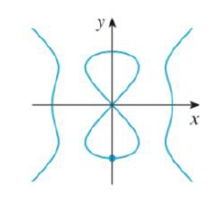Chapter 3.5, Problem 32E

Chapter
Section
Textbook Problem

Use implicit differentiation to find an equation of the tangent line to the curve at the given point.32. y2(y2 – 4) = x2(x2 –5), (0. –2), (devil’s curve)To determine

To find: The equation of the tangent line to the given equation at the point.

Explanation

Given:

The curve is y2(y24)=x2(x25).

The point is (0,2).

Derivative rules:

(1) Chain rule: If y=f(u) and u=g(x)  are both differentiable function, then dydx=dydududx.

(2) Product rule: ddx(f(x)g(x))=f(x)ddx(g(x))+g(x)ddx(f(x))

Formula used:

The equation of the tangent line at (x1,y1) is, yy1=m(xx1) (1)

Where, m is the slope of the tangent line at (x1,y1) and m=dydx|x=x1,y=y1.

Calculation:

Obtain the equation of the tangent line to the equation at the point.

Rewrite the given equation as follows,

y2(y24)=x2(x25)y44y2=x45x2

Differentiate the equation implicitly with respect to x,

ddx(y44y2)=ddx(x45x2)ddx(y4)4ddx(y2)=ddx(x4)5ddx(x2)ddx(y4)4ddx(y2)=4x210x<

Still sussing out bartleby?

Check out a sample textbook solution.

See a sample solution

The Solution to Your Study Problems

Bartleby provides explanations to thousands of textbook problems written by our experts, many with advanced degrees!

Get Started

Find all possible real solutions of each equation in Exercises 3144. x3x23x+3=0

Finite Mathematics and Applied Calculus (MindTap Course List)

In Exercises 4143, find the distance between the two points. 43. (12,3) and (12,23)

Applied Calculus for the Managerial, Life, and Social Sciences: A Brief Approach

Evaluate the integral. 37. 0/4tan3sec2d

Single Variable Calculus: Early Transcendentals

The angle θ at the right has cosine:

Study Guide for Stewart's Multivariable Calculus, 8th

For the area of the shaded region, which integral form, dx or dy, should be used? a) dx b) dy c) Either...

Study Guide for Stewart's Single Variable Calculus: Early Transcendentals, 8th